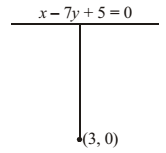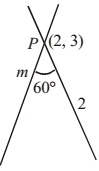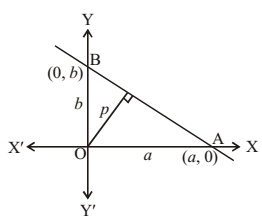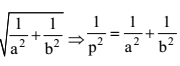## NCERT Solutions for Class 11 Maths Chapter 10 Straight Lines Exercise 10.3

If you are facing trouble finding the correct NCERT Solutions of Chapter 10 Straight Lines Exercise 10.3 then you we have provided these for you here. It will help you in finding the NCERT Solutions for Class 11 Maths difficult questions. The solutions provided here are updated as per the latest pattern of CBSE which will improve your problem solving skills.1. Reduce the following equations into slope intercept form and find their slopes and intercepts on the axes.
(i) x + 7y = 0
(ii) 6x + 3y – 5 = 0
(iii) y = 0

(i) x + 7y = 0 or y = - (1/7) x + 0
∴ Slope = -(1/7)  and y-intercept = 0

(ii) 6x + 3y – 5 = 0 or 3y = – 6x + 5
y = – 2x + 5/3
∴ Slope = – 2 and y-intercept = 5/3

(iii) y = 0 or y = 0.x + 0
∴ Slope = 0, y-intercept = 0.

2. Reduce the following equations into intercepts form and their intercepts on the axes.
(i) 3x + 2y – 12 = 0
(ii) 4x – 3y = 6
(iii) 3y + 2 = 0

(i) 3x + 2y – 12 = 0
or 3x + 2y = 12
or 3x/12 + 2y/12 = 1
or x/4 + y/6 = 1 (intercepts form)
where a = 4, b = 6

(ii) 4x + 3y = 6
4x/6 – 3y/6 = 1
x/3/2 + y/-2 = 1 (intercepts form)
where a = 3/2, b = -2

(iii) 3y + 2 = 0
3y = 2
3y/-2 = 1 => y/-2/3 = 1
Now b = -(2/3) and no intercept along x- axis.

3. Reduce the following equations into normal form. Find their perpendicular distance from the origin and angle between perpendicular and the positive x-axis.
(i) x – √3y + 8 = 0
(ii) y – 2 = 0
(iii) x – y = 4

(i) x – √3y + 8 = 0
-x + √3y = 8
Now a = 1, b = √3
Here √a2 + b2 = √1 + 3 = √4 = 2
Thus, -(x/2) + √3/2 y = 8/2
-(x/2) + √3/2 y = 4
Or x cos 120° + y sin 120° = 4 (normal form)
Where p = 4 and ω = 120°
(ii) y – 2 = 0
y = 2
now a = 0, b = 1
here √a2 + b2 = √0 + 1 = 1
thus, 0x + 1y = 2
or x cos 90° + ysin 90° = 2 (normal form)
where ω = 90° and p = 2
(iii) x – y = 4
Now a = 1, b = -1
Here, √a2 + b2 = √1 + 1 = √2
Thus, 1/√2 x – 1/√2y = 4
Or xcos 315° + ysin(315°) = 4(normal form)
Where ω = 315° and p = 4

4. Find the distance of the point (–1, 1) from the line 12(x + 6) = 5 (y – 2)5. Find the points on the x-axis, whose distances from the line x/3 + y/4 = 1 are 4 units.

The given line is x/3 + y/4 = 1
Multiplying by 12
4x + 3y = 12 or 4x + 3y – 12 = 0
Any point on the x-axis is (x, 0)
Perpendicular distance from (x, 0) to the given line
(4x – 12)/√42 + 32 = 4
Or | 4x - 12 |/√25 = 4 or | 4x - 12 | = 5 × 4 = 20
Taking (+ve)sign
4x – 12 = 20 or 4x = 20 + 12 = 32
=> x = 32/4 = 8
Taking (-ve) sign 4x – 12 = -20
4x = -20 + 12 = -8
x = -2
∴ required points on the x – axis are (8, 0) and (-2, 0)

6. Find the distance between parallel lines
(i) 15x + 8y – 34 = 0 and 15x + 8y + 31 = 0
(ii) l(x + y) + p = 0 and l(x + y) – r = 0.

(i) One of the lines is 15x + 8y – 34 = 0
Putting y = 0, 15x = 34 or x = 34/15
∴ The point (34/15, 0) lies on
15x + 8y – 34 = 0
∴ perpendicular distance from (34/15 , 0)
to 15x + 8y + 31 = 0 is
= |15 . 34/15 + 0 + 31|/√152 + 82 =| 34 + 31/√225 + 64
= 65/√289 = 65/17
(ii) l(x + y) + p = 0
lx + ly – r = 0
Now A = l, B = l, C1 = p, C2 = –r
Distance between parallel lines
d = |C1 – C2|/√A2 + B2
= |p + r|/√l2 + l2 = |p + r|/√2 l = 1/√2 |(p + r)/l|

7. Find equation of the line parallel to the line 3x – 4y + 2 = 0 and passing through the point (–2, 3).

The given line is 3x – 4y + 2 = 0
Slope of the line = 3/4
Let equation of the parallel line to the given line
3x – 4y + 2 is 3x – 4y + λ = 0
Required line passing through point (–2, 3)
∴ 3 × (–2) – 4 × 3 + λ = 0
=>  – 6 – 12 + λ = 0
∴  λ = 18
∴ Required equation is 3x – 4y + 18 = 0

8. Find the equation of the line perpendicular to the line x – 7y + 5 = 0 and having x-intercept 3.

Equation of required line is 7x + y + λ = 0It passes through the point (3, 0)
∴ 7 × 3 + 0 + λ = 0
λ=-21
∴ Required equation is 7x + y – 21 = 0.

9. Find the angles between the lines √3x + y = 1 and x + √3y =1.

The first line is √3x +y = 1 or y = -√3 . x + 1
The slope m1 of the line √3x + y = 1 is - √3
or, m1 = - √3
The other line is
x + √3y = 1 => y = -(1/√3) x + 1/√3
The slope m2 of the line x + √3y = 1 is –(1/√3)
∴ m2 = -(1/√3)
if θ is the angle between two lines, then
tan θ =tanθ = 2/√3 . 1/2 = 1/√3 => θ = π/6

10. The line through the points (h, 3) and (4, 1) intersects the line 7x – 9y – 19 = 0 at right angle. Find the value of h.

Slope of the line PQ passing through P(h, 3) and Q (4, 1) is 2/(h – 4)
Slope of the line AB 7x – 9y – 19 = 0 is 7/9.The lines AB and PQ are perpendicular to each other
∴ Product of their slopes = –1
Or 2/(h – 4) × 7/9 = -1    ∴ 14 = -9(h – 4)
Or 9h = 36 – 14 or 9h = 22
Or h = 22/9.

11. Prove that the line through the point (x1, y 1) and parallel to the line Ax + By + C = 0 is A(x – x1) + B(y – y 1 )= 0.

The given line is Ax + By + C = 0
or y = -(A/B)x – C/BSlope of the parallel line to the line
Ax + By + C = 0 is –A/B
The parallel line passes through (x 1, y 1)
∴ Equation of the parallel line passing through (x 1, y 1) is
∴  y – y1 = -(A/B)(x – x1)
=> B(y – y1) = -A(x – x1)
A(x – x1) + B(y – y1) = 0 Hence proved.

12. Two lines passing through the point (2, 3) intersects each other at an angle 60°. If slope of one line is 2, find equation of the other line.

Let m be the slope of the other line and given slope of one line = 2
and angle between them = 60°
∴ tan 60 = ± (m – 2)/(1 + 2m)
=> (m – 2)/(1 + 2m) = ± √3For +ve sign,
m – 2 = √3(1 + 2m) = √3 + 2√3m
(2√3 – 1)m = -2-√3
∴ m = -((2 + √3)/(2√3 – 1))
Equation of the line passing through P(2, 3) with slope m is
y – 3 = -((2 + √3)/(2√3 – 1))( x – 2)
=> (2√3 – 1)y – 3(2√3 – 1)
= -(2 + √3)x + 2(2 + √3)
=> (2√3 – 1)y – 6√3 + 3
= -(2 + √3)x + 4 + 2√3
=> (2 + √3)x + (2√3 – 1)y – 6√3 + 3 – 4 – 2√3 = 0
=> (2 + √3)x + (2√3 – 1)y – 8√3 – 1 = 0
For –ve sign (m – 2)/(1 + 2m) = - √3
=> m – 2 = -√3(1 + 2m) = -√3 – 2√3m
(2√3 + 1)m = 2 – √3
∴ m = - ((2 – √3)/(1 + 2√3))
Equation of the line passing through P(2, 3) with slope m is
y – 3 = -((2 – √3)/(1 + 2√3))(x – 2)
=> (1 + 2√3)y – 3(1 + 2√3)
= (2 – √3)x – 2(2 – √3)
=> (1 + 2√3)y – 3 – 6√3 = (2 – √3)x – 4+ 2√3
=> (2 – √3)x – (1 + 2√3)y – 4 + 2√3 + 3 + 6√3 = 0
=> (2 – √3)x – (1 + 2√3)y – 1 + 8√3 = 0
∴ Required lines are
(√3 + 2)x + (2√3 – 1)y – 8√3 – 1 = 0
And (2 – √3)x – (1 + 2√3)y + 8√3 – 1 = 0.

13. Find the equation of the right bisector of the line segment joining the points (3, 4) and (–1, 2).

Slope of the line joining the points A(–1, 2) and B(3, 4)
= (4 – 2)/(3 + 1) = 2/4 = 1/2Right bisector PQ is perpendicular to AB
∴ Slope of PQ = – 2
middle point of AB is ((-1 + 3)/2 , (2 + 4)/2 ) i.e. (1, 3)
Right bisector passes through M(1, 3)
Equation of right bisector PQ is y – 3
= – 2 (x – 1) = –2x + 2
=>  2x + y – 3 – 2 = 0 Þ 2x + y – 5 = 0.

14. Find the coordinates of the foot of perpendicular from the point (–1, 3) to the line 3x –4y – 16 = 0.

The equation of the given line is
3x – 4y – 16 = 0
Let the equation of a line perpendicular to the given line is 4x + 3y + k = 0, where k is a constant .If this line passes through the point (–1, 3), then
– 4 + 9 + k = 0 => k = –5
∴ The equation of a line passing through the point (–1, 3) and perpendicular to the given line is
4x + 3y – 5 = 0
∴ The required point of the foot of the perpendicular is the point of the intersection of the lines
3x – 4y – 16 = 0 … (i)
and 4x + 3y – 5 = 0 … (ii)
Solving (i) and (ii) by cross-multiplication, we have
x/(20 + 48) = y/(-64 + 15) = 1/(9 + 16)
=> x/68 = y/-49 = 1/25 => x = 68/25 , y = -(49/25)
∴ The required point is (68/25 , -49/25).

15. The perpendicular from the origin to the line y = mx + c meets it at the point (– 1, 2). Find the values of m and c.

Let the perpendicular OM is drawn from the origin to AB.M is the foot of the perpendicular
Slope of OM = (2 – 0)/(-1 – 0) = 2/-1 ;
Slope of AB = m
OM ⊥ AB ∴ m × (-2) = -1  ∴ m = 1/2
M(–1, 2) lies on AB whose equation is
y = mx + c or y = 1/2 x + c
2 = 1/2 × (-1) + c => c = 2 + 1/2 = 5/2
∴ m = 1/2 or c = 5/2.

16. If p and q are the lengths of perpendiculars from the origin to the lines x cos θ – y sin θ = k cos 2θ and x sec θ + y cosec θ = k respectively, prove that p2+ 4q2= k2 .

The perpendicular distance from the origin to the line x cosθ – y sinθ = k cos2θ …(i)
p = (kcos 2θ)/cos2θ + sin2θ = kcos 2θ,
the other line is x secθ + y cosecθ = k
or x/cosθ + y/sinθ = k
xsinθ + ycosθ = ksinθ cosθ
xsinθ + ycosθ = k/2 sin 2θ …….(ii)
∴ the perpendicular distance q from the origin to the line (ii)
q = (k/2 sin 2θ)/(sin2θ+ cos 2θ) = k/2 sin 2θ
now p2 + 4q2 = k2 cos22 + 4(k/2 sin 2)2
=> p2 + 4q2 = k2 cos2 2θ + 4. K2/4 sin2 2θ
p2 + 4q2 = k2(cos22θ + sin 22θ),
Hence, p2 + 4q2 = k2

17. In the triangle ABC with vertices A(2, 3), B(4, –1) and C(1, 2), find the equation and length of altitude from the vertex A.

The vertices of △ABC are A(2, 3), B(4, –1) and C(1, 2) and AM is the altitude.
Slope of BC = (2 + 1)/(1 – 4) = 3/-3 = -1
∴ Slope of altitude AM = 1
Now, altitude passes through A(2, 3) and has the slope 1.∴ Equation of AM is y – 3 = (x – 2)
x – y + 3 – 2 = 0
or y – x = 1
Equation of BC passing through B(4, –1) and C(1, 2)
y + 1 = (2 – 4)/(1 + 1) (x – 4) => y + 1 = -2/2 (x – 4)
y + 1 = -(x – 4)
or x + y = 3 or x + y – 3 = 0
∴ length of altitude = AM
= perpendicular distance from A(2, 3) to BC.
= (2 + 3 – 3)/(√12 + 12) = 2/√2 = √2
∴ Length of altitude = √2
Equation of altitude is y – x = 1 and length of altitude = √2.

18. If p is the length of perpendicular from the origin to the line whose intercepts on the axes are a and b, then show that 1/p2 = 1/a2 + 1/b2.p ==> 1/p =